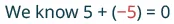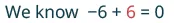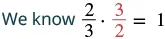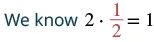Prealgebra

# 7.4Properties of Identity, Inverses, and Zero

Prealgebra7.4 Properties of Identity, Inverses, and Zero

### Learning Objectives

By the end of this section, you will be able to:
• Recognize the identity properties of addition and multiplication
• Use the inverse properties of addition and multiplication
• Use the properties of zero
• Simplify expressions using the properties of identities, inverses, and zero

### Be Prepared 7.4

Before you get started, take this readiness quiz.

1. Find the opposite of $−4.−4.$
If you missed this problem, review Example 3.3.
2. Find the reciprocal of $52.52.$
If you missed this problem, review Example 4.29.
3. Multiply: $3a5·92a.3a5·92a.$
If you missed this problem, review Example 4.27.

### Recognize the Identity Properties of Addition and Multiplication

What happens when we add zero to any number? Adding zero doesn’t change the value. For this reason, we call $00$ the additive identity.

For example,

$13+0−14+00+(−3x) 13−14−3x 13+0−14+00+(−3x) 13−14−3x$

What happens when you multiply any number by one? Multiplying by one doesn’t change the value. So we call $11$ the multiplicative identity.

For example,

$43·1−27·11·6y543−276y543·1−27·11·6y543−276y5$

### Identity Properties

The identity property of addition: for any real number $a,a,$

$a+0=a0+a=a0 is called the additive identitya+0=a0+a=a0 is called the additive identity$

The identity property of multiplication: for any real number $aa$

$a·1=a1·a=a1 is called the multiplicative identitya·1=a1·a=a1 is called the multiplicative identity$

### Example 7.33

Identify whether each equation demonstrates the identity property of addition or multiplication.

1. $7+0=77+0=7$

2. $−16(1)=−16−16(1)=−16$

### Try It 7.65

Identify whether each equation demonstrates the identity property of addition or multiplication:

$23+0=2323+0=23$$−37(1)=−37.−37(1)=−37.$

### Try It 7.66

Identify whether each equation demonstrates the identity property of addition or multiplication:

$1·29=291·29=29$$14+0=14.14+0=14.$

### Use the Inverse Properties of Addition and Multiplication

 What number added to 5 gives the additive identity, 0? $5+_____=05+_____=0$What number added to −6 gives the additive identity, 0? $−6+_____=0−6+_____=0$Notice that in each case, the missing number was the opposite of the number.

We call $−a−a$ the additive inverse of $a.a.$ The opposite of a number is its additive inverse. A number and its opposite add to $0,0,$ which is the additive identity.

What number multiplied by $2323$ gives the multiplicative identity, $1?1?$ In other words, two-thirds times what results in $1?1?$

 $23·___=123·___=1$What number multiplied by $22$ gives the multiplicative identity, $1?1?$ In other words two times what results in $1?1?$

 $2·___=12·___=1$Notice that in each case, the missing number was the reciprocal of the number.

We call $1a1a$ the multiplicative inverse of $a(a≠0).a(a≠0).$ The reciprocal of a number is its multiplicative inverse. A number and its reciprocal multiply to $1,1,$ which is the multiplicative identity.

We’ll formally state the Inverse Properties here:

### Inverse Properties

Inverse Property of Addition for any real number $a,a,$

$a+(−a)=0−ais the additive inverse ofa.a+(−a)=0−ais the additive inverse ofa.$

Inverse Property of Multiplication for any real number $a≠0,a≠0,$

$a·1a=11ais the multiplicative inverse ofa.a·1a=11ais the multiplicative inverse ofa.$

### Example 7.34

Find the additive inverse of each expression: $1313$ $−58−58$ $0.60.6$.

### Try It 7.67

Find the additive inverse: $1818$ $7979$ $1.21.2$.

### Try It 7.68

Find the additive inverse: $4747$ $713713$ $8.48.4$.

### Example 7.35

Find the multiplicative inverse: $99$ $−19−19$ $0.90.9$.

### Try It 7.69

Find the multiplicative inverse: $55$ $−17−17$ $0.30.3$.

### Try It 7.70

Find the multiplicative inverse: $1818$ $−45−45$ $0.60.6$.

### Use the Properties of Zero

We have already learned that zero is the additive identity, since it can be added to any number without changing the number’s identity. But zero also has some special properties when it comes to multiplication and division.

#### Multiplication by Zero

What happens when you multiply a number by $0?0?$ Multiplying by $00$ makes the product equal zero. The product of any real number and $00$ is $0.0.$

### Multiplication by Zero

For any real number $a,a,$

$a·0=00·a=0a·0=00·a=0$

### Example 7.36

Simplify: $−8·0−8·0$ $512·0512·0$ $0(2.94)0(2.94)$.

### Try It 7.71

Simplify: $−14·0−14·0$ $0·230·23$ $(16.5)·0.(16.5)·0.$

### Try It 7.72

Simplify: $(1.95)·0(1.95)·0$ $0(−17)0(−17)$ $0·54.0·54.$

#### Dividing with Zero

What about dividing with $0?0?$ Think about a real example: if there are no cookies in the cookie jar and three people want to share them, how many cookies would each person get? There are $00$ cookies to share, so each person gets $00$ cookies.

$0÷3=00÷3=0$

Remember that we can always check division with the related multiplication fact. So, we know that

$0÷3=0because0·3=0.0÷3=0because0·3=0.$

### Division of Zero

For any real number $a,a,$ except $0,0a=00,0a=0$ and $0÷a=0.0÷a=0.$

Zero divided by any real number except zero is zero.

### Example 7.37

Simplify: $0÷50÷5$ $0−20−2$ $0÷780÷78$.

### Try It 7.73

Simplify: $0÷110÷11$ $0−60−6$ $0÷3100÷310$.

### Try It 7.74

Simplify: $0÷830÷83$ $0÷(−10)0÷(−10)$ $0÷12.750÷12.75$.

Now let’s think about dividing a number by zero. What is the result of dividing $44$ by $0?0?$ Think about the related multiplication fact. Is there a number that multiplied by $00$ gives $4?4?$

$4÷0=___means___·0=44÷0=___means___·0=4$

Since any real number multiplied by $00$ equals $0,0,$ there is no real number that can be multiplied by $00$ to obtain $4.4.$ We can conclude that there is no answer to $4÷0,4÷0,$ and so we say that division by zero is undefined.

### Division by Zero

For any real number $a,a0,a,a0,$ and $a÷0a÷0$ are undefined.

Division by zero is undefined.

### Example 7.38

Simplify: $7.5÷07.5÷0$ $−320−320$ $49÷049÷0$.

### Try It 7.75

Simplify: $16.4÷016.4÷0$ $−20−20$ $15÷015÷0$.

### Try It 7.76

Simplify: $−50−50$ $96.9÷096.9÷0$ $415÷0415÷0$

We summarize the properties of zero.

### Properties of Zero

Multiplication by Zero: For any real number $a,a,$

$a·0=00·a=0The product of any number and 0 is 0.a·0=00·a=0The product of any number and 0 is 0.$

Division by Zero: For any real number $a,a≠0a,a≠0$

$0a=00a=0$ Zero divided by any real number, except itself, is zero.

$a0a0$ is undefined. Division by zero is undefined.

### Simplify Expressions using the Properties of Identities, Inverses, and Zero

We will now practice using the properties of identities, inverses, and zero to simplify expressions.

### Example 7.39

Simplify: $3x+15−3x.3x+15−3x.$

### Try It 7.77

Simplify: $−12z+9+12z.−12z+9+12z.$

### Try It 7.78

Simplify: $−25u−18+25u.−25u−18+25u.$

### Example 7.40

Simplify: $4(0.25q).4(0.25q).$

### Try It 7.79

Simplify: $2(0.5p).2(0.5p).$

### Try It 7.80

Simplify: $25(0.04r).25(0.04r).$

### Example 7.41

Simplify: $0n+50n+5$, where $n≠−5n≠−5$.

### Try It 7.81

Simplify: $0m+70m+7$, where $m≠−7m≠−7$.

### Try It 7.82

Simplify: $0d−40d−4$, where $d≠4d≠4$.

### Example 7.42

Simplify: $10−3p0.10−3p0.$

### Try It 7.83

Simplify: $18−6c0.18−6c0.$

### Try It 7.84

Simplify: $15−4q0.15−4q0.$

### Example 7.43

Simplify: $34·43(6x+12).34·43(6x+12).$

### Try It 7.85

Simplify: $25·52(20y+50).25·52(20y+50).$

### Try It 7.86

Simplify: $38·83(12z+16).38·83(12z+16).$

All the properties of real numbers we have used in this chapter are summarized in Table 7.1.

Commutative Property
If a and b are real numbers then… $a+b=b+aa+b=b+a$ $a·b=b·aa·b=b·a$
Associative Property
If a, b, and c are real numbers then… $(a+b)+c=a+(b+c)(a+b)+c=a+(b+c)$ $(a·b)·c=a·(b·c)(a·b)·c=a·(b·c)$
Identity Property $00$ is the additive identity $11$ is the multiplicative identity
For any real number a, $a+0=a0+a=aa+0=a0+a=a$ $a·1=a1·a=aa·1=a1·a=a$
Inverse Property $−a−a$is the additive inverse of $aa$ $a,a≠0a,a≠0$
$1/a1/a$ is the multiplicative inverse of $aa$
For any real number a, $a+(−a)=0a+(−a)=0$ $a·1a=1a·1a=1$
Distributive Property
If $a,b,ca,b,c$ are real numbers, then $a(b+c)=ab+aca(b+c)=ab+ac$
Properties of Zero
For any real number a,
$a⋅0=00⋅a=0a⋅0=00⋅a=0$
For any real number $a,a≠0a,a≠0$ $0a=00a=0$
$a0a0$ is undefined
Table 7.1 Properties of Real Numbers

### Section 7.4 Exercises

#### Practice Makes Perfect

Recognize the Identity Properties of Addition and Multiplication

In the following exercises, identify whether each example is using the identity property of addition or multiplication.

158.

$101 + 0 = 101 101 + 0 = 101$

159.

$3 5 ( 1 ) = 3 5 3 5 ( 1 ) = 3 5$

160.

$−9 · 1 = −9 −9 · 1 = −9$

161.

$0 + 64 = 64 0 + 64 = 64$

Use the Inverse Properties of Addition and Multiplication

In the following exercises, find the multiplicative inverse.

162.

$8 8$

163.

$14 14$

164.

$−17 −17$

165.

$−19 −19$

166.

$7 12 7 12$

167.

$8 13 8 13$

168.

$− 3 10 − 3 10$

169.

$− 5 12 − 5 12$

170.

$0.8 0.8$

171.

$0.4 0.4$

172.

$−0.2 −0.2$

173.

$−0.5 −0.5$

Use the Properties of Zero

In the following exercises, simplify using the properties of zero.

174.

$48 · 0 48 · 0$

175.

$0 6 0 6$

176.

$3 0 3 0$

177.

$22 · 0 22 · 0$

178.

$0 ÷ 11 12 0 ÷ 11 12$

179.

$6 0 6 0$

180.

$0 3 0 3$

181.

$0 ÷ 7 15 0 ÷ 7 15$

182.

$0 · 8 15 0 · 8 15$

183.

$( −3.14 ) ( 0 ) ( −3.14 ) ( 0 )$

184.

$5.72 ÷ 0 5.72 ÷ 0$

185.

$1 10 0 1 10 0$

Simplify Expressions using the Properties of Identities, Inverses, and Zero

In the following exercises, simplify using the properties of identities, inverses, and zero.

186.

$19 a + 44 − 19 a 19 a + 44 − 19 a$

187.

$27 c + 16 − 27 c 27 c + 16 − 27 c$

188.

$38 + 11 r − 38 38 + 11 r − 38$

189.

$92 + 31 s − 92 92 + 31 s − 92$

190.

$10 ( 0.1 d ) 10 ( 0.1 d )$

191.

$100 ( 0.01 p ) 100 ( 0.01 p )$

192.

$5 ( 0.6 q ) 5 ( 0.6 q )$

193.

$40 ( 0.05 n ) 40 ( 0.05 n )$

194.

$0r+200r+20$, where $r≠−20r≠−20$

195.

$0s+130s+13$, where $s≠−13s≠−13$

196.

$0u−4.990u−4.99$, where $u≠4.99u≠4.99$

197.

$0v−65.10v−65.1$, where $v≠65.1v≠65.1$

198.

$0÷(x−12)0÷(x−12)$, where $x≠12x≠12$

199.

$0÷(y−16)0÷(y−16)$, where $y≠16y≠16$

200.

$32−5a032−5a0$, where $32−5a≠032−5a≠0$

201.

$28−9b028−9b0$, where $28−9b≠028−9b≠0$

202.

$2.1+0.4c02.1+0.4c0$, where $2.1+0.4c≠02.1+0.4c≠0$

203.

$1.75+9f01.75+9f0$, where $1.75+9f≠01.75+9f≠0$

204.

$(34+910m)÷0(34+910m)÷0$, where $34+910m≠034+910m≠0$

205.

$(516n−37)÷0(516n−37)÷0$, where $516n−37≠0516n−37≠0$

206.

$9 10 · 10 9 ( 18 p − 21 ) 9 10 · 10 9 ( 18 p − 21 )$

207.

$5 7 · 7 5 ( 20 q − 35 ) 5 7 · 7 5 ( 20 q − 35 )$

208.

$15 · 3 5 ( 4 d + 10 ) 15 · 3 5 ( 4 d + 10 )$

209.

$18 · 5 6 ( 15 h + 24 ) 18 · 5 6 ( 15 h + 24 )$

#### Everyday Math

210.

Insurance copayment Carrie had to have $55$ fillings done. Each filling cost $80.80.$ Her dental insurance required her to pay $20%20%$ of the cost. Calculate Carrie’s cost

1. by finding her copay for each filling, then finding her total cost for $55$ fillings, and

2. by multiplying $5(0.20)(80).5(0.20)(80).$

3. Which of the Properties of Real Numbers did you use for part (b)?

211.

Cooking time Helen bought a $24-pound24-pound$ turkey for her family’s Thanksgiving dinner and wants to know what time to put the turkey in the oven. She wants to allow $2020$ minutes per pound cooking time.

1. Calculate the length of time needed to roast the turkey by multiplying $24·2024·20$ to find the number of minutes and then multiplying the product by $160160$ to convert minutes into hours.

2. Multiply $24(20·160).24(20·160).$

3. Which of the Properties of Real Numbers allows you to multiply $24(20·160)24(20·160)$ instead of $(24·20)160?(24·20)160?$

#### Writing Exercises

212.

In your own words, describe the difference between the additive inverse and the multiplicative inverse of a number.

213.

How can the use of the properties of real numbers make it easier to simplify expressions?

#### Self Check

After completing the exercises, use this checklist to evaluate your mastery of the objectives of this section.

On a scale of 1–10, how would you rate your mastery of this section in light of your responses on the checklist? How can you improve this?

Order a print copy

As an Amazon Associate we earn from qualifying purchases.